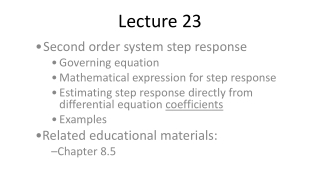DownloadDownload PresentationLecture 23

# Lecture 23

Télécharger la présentation## Lecture 23

- - - - - - - - - - - - - - - - - - - - - - - - - - - E N D - - - - - - - - - - - - - - - - - - - - - - - - - - -
##### Presentation Transcript

1. Lecture 23 Second order system step response Governing equation Mathematical expression for step response Estimating step response directly from differential equation coefficients Examples Related educational materials: Chapter 8.5

2. Second order system step response • Governing equation in “standard” form: • Initial conditions: • We will assume that the system is initially “relaxed”

3. Second order system step response – continued • We will concentrate on the underdamped response: • Looks like the natural response superimposed with a step function

4. Step response parameters • We would like to get an approximate, but quantitative estimate of the step response, without explicitly determining y(t) • Several step response parameters are directly related to the coefficients of the governing differential equation • These relationships can also be used to estimate the differential equation from a measured step response • Model parameter estimation

5. Second order system step response – plot

6. Steady-state response • Input-output equation: • As t, circuit parameters become constant so: • Circuit DC gain:

7. On previous slide, note that DC gain can be determined directly from circuit.

8. Rise time • Rise time is the time required for the response to get from 10% to 90% of yss • Rise time is closely related to the natural frequency:

9. Maximum overshoot, MP • MP is a measure of the maximum response value • MP is often expressed as a percentage of yss and is related directly to the damping ratio:

10. Maximum overshoot – continued • For small values of damping ratio, it is often convenient to approximate the previous relationship as:

11. Example 1 • Determine the maximum value of the current, i(t), in the circuit below

12. In previous slide, outline overall approach: • Need MP, and steady-state value • Need damping ratio to get MP • Need natural frequency to get damping ratio • Need to determine differential equation

13. Step 1: Determine differential equation

14. Step 2: Identify n, , and steady-state current • Governing equation:

15. Step 3: Determine maximum current • Damping ratio,  = 0.54 • Steady-state current,

16. Example 2 • Determine the differential equation governing iL(t) and the initial conditions iL(0+) and vc(0+)

17. Example 2 – differential equation, t>0

18. Example 2 – initial conditions

19. Example 3 – model parameter estimation The differential equation governing a system is known to be of the form: When a 10V step input is applied to the system, the response is as shown. Estimate the differential equation governing the system.

20. Example 3 – find tr, MP, yss from plot

21. Example 3 – find differential equation • From plot, we determined: • MP  0.25 • tr 0.05 • yss  0.002

22. Example 4 – Series RLC circuit • MP 100%, n = 100,000 rad/sec (16KHz)